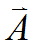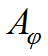﻿ Technical Notes > Applications in Electromagnetics > Magnetostatics > A Magnet Coil in 2D Cylindrical Coordinates

# A Magnet Coil in 2D Cylindrical Coordinates

Navigation:  Technical Notes > Applications in Electromagnetics > Magnetostatics >

# A Magnet Coil in 2D Cylindrical CoordinatesAs a first example, we will calculate the magnetic field created by a coil, using 2D cylindrical (r,z) geometry.  We will apply current only in the azimuthal direction, so the only nonzero component ofwill be the azimuthal component.  With only a single component normal to the computational plane, the gauge condition is automatically satisfied, since.

In the descriptor which follows, note that we have chosen to align the cylindrical axis with the horizontal plot axis. FlexPDE uses a right-hand coordinate system, so in this case positiveis outward from the plot page.

#### Descriptor 2.1: Magnet_Coil.pde

Title 'AXI-SYMMETRIC MAGNETIC FIELD'

Coordinates

xcylinder(Z,R)

Variables

Aphi   { azimuthal component of the vector potential }

Definitions

mu = 1             { the permeability }

J = 0                 { global source term defaults to zero }

current = 10       { the source value in the coil }

Br = -dz(Aphi)     { definitions for plots }

Bz = dr(r*Aphi)/r

Equations

Curl(curl(Aphi)/mu) = J

Boundaries

Region 1

start(-10,0)

value(Aphi) = 0     { specify A=0 along axis }

line to (10,0)

value(Aphi) = 0     { H x n = 0 on distant sphere }

arc(center=0,0) angle 180 to close

Region 2

J = current         { redefine source value }

start (-0.25,1)

line to (0.25,1) to (0.25,1.5)

to (-0.25,1.5) to close

Monitors

contour(Bz) zoom(-2,0,4,4) as 'FLUX DENSITY B'

contour(Aphi) as 'Potential'

Plots

grid(z,r)

contour(Bz) as 'FLUX DENSITY B'

contour(Bz) zoom(-2,0,4,4) as 'FLUX DENSITY B'

elevation(Aphi, dr(Aphi), Aphi/r, Bz)

from (0,0) to (0,1) as 'Near Axis'

vector(Bz,Br) as 'FLUX DENSITY B'

vector(Bz,Br) zoom(-2,0,4,4) as 'FLUX DENSITY B'

contour(Aphi) as 'MAGNETIC POTENTIAL'

contour(Aphi) zoom(-2,0,4,4) as 'MAGNETIC POTENTIAL'

surface(Aphi) as 'MAGNETIC POTENTIAL'

viewpoint (-1,1,30)

End